# Capillary waves

## Thermal capillary waves

Thermal capillary waves are oscillations of an interface which are thermal in origin. These take place at the molecular level, where only the surface tension contribution is relevant.

Capillary wave theory (CWT) is a classic account of how thermal fluctuations distort an interface (Ref. 1). It starts from some intrinsic surface that is distorted. By performing a Fourier analysis treatment, normal modes are easily found. Each contributes a energy proportional to the square of its amplitude; therefore, according to classical statistical mechanics, equipartition holds, and the mean energy of each mode will be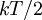$kT/2$. Surprisingly, this result leads to a divergent surface (the width of the interface is bound to diverge with its area) (Ref 2). This divergence is nevertheless very mild: even for displacements on the order of meters the deviation of the surface is comparable to the size of the molecules. Moreover, the introduction of an external field removes the divergence: the action of gravity is sufficient to keep the width fluctuation on the order of one molecular diameter for areas larger than about 1 mm2 (Ref. 2).

Recently, a procedure has been proposed to obtain a molecular intrinsic surface from simulation data (Ref. 3). The density profiles obtained from this surface are, in general, quite different from the usual mean density profiles.

## Gravity-capillary waves

These are ordinary waves excited in an interface, such as ripples on a water surface. Their dispersion relation reads, for waves on the interface between two fluids of infinite depth: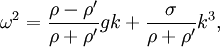$\omega^2=\frac{\rho-\rho'}{\rho+\rho'}gk+\frac{\sigma}{\rho+\rho'}k^3,$

where ω is the angular frequency, g the acceleration due to gravity, σ the surface tension, ρ and ρ‘ the mass density of the two fluids (ρ > ρ‘) and k the wavenumber.

### Derivation

This is a sketch of the derivation of the general dispersion relation, see Ref. 4 for a more detailed description. The problem is unfortunately a bit complex. As Richard Feynman put it (Ref. 5):

...[water waves], which are easily seen by everyone and which are used as an example of waves in elementary courses... are the worst possible example... they have all the complications that waves can have

#### Defining the problem

Three contributions to the energy are involved: the surface tension, gravity, and hydrodynamics. The part due to gravity is the simplest: integrating the potential energy density due to gravity,$\rho g z$ from a reference height to the position of the surface,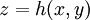$z=h(x,y)$: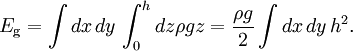$E_\mathrm{g}= \int dx\, dy\, \int_0^h dz \rho g z = \frac{\rho g}{2} \int dx\, dy\, h^2.$

(For simplicity, we are neglecting the density of the fluid above, which is often acceptable.)

An increase in area of the surface causes a proportional increase of energy: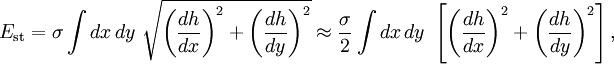$E_\mathrm{st}= \sigma \int dx\, dy\ \sqrt{\left( \frac{dh}{dx} \right)^2+\left( \frac{dh}{dy} \right)^2} \approx \frac{\sigma}{2} \int dx\, dy\ \left[ \left( \frac{dh}{dx} \right)^2+\left( \frac{dh}{dy} \right)^2 \right],$

where the fist equality is the area in this (de Monge) representation, and the second applies for small values of the derivatives (surfaces not too rough).

The last contribution involves the kinetic energy of the fluid: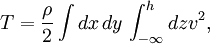$T= \frac{\rho}{2} \int dx\, dy\, \int_{-\infty}^h dz v^2,$

where$v$ is the module of the velocity field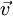$\vec{v}$.

#### Wave solutions

Let us try separation of variables: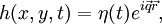$h(x,y,t)=\eta(t)e^{i\vec{q}\vec{r}},$

where$\vec{q}=(q_x,q_y)$ is a two dimensional wave number vector, and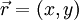$\vec{r}=(x,y)$ the position. In this case,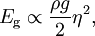$E_\mathrm{g} \propto \frac{\rho g}{2} \eta^2,$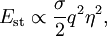$E_\mathrm{st} \propto \frac{\sigma}{2} q^2 \eta^2,$

where a factor of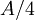$A/4$ that will appear every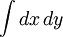$\int dx\, dy\$ integration is dropped for convenience.

To tackle the kinetic energy, suppose the fluid is incompressible and its flow is irrotational (often, sensible approximations) - the flow will then be potential: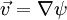$\vec{v}=\nabla\psi$, and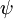$\psi$ is a potential (scalar field) which must satisfy Laplace equation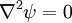$\nabla^2\psi=0$. If we try try separation of variables with the potential: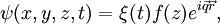$\psi(x,y,z,t)=\xi(t) f(z) e^{i\vec{q}\vec{r}},$

with some function of time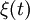$\xi(t)$, and some function of vertical component (height)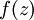$f(z)$ Laplace equation then requires on the later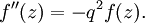$f''(z)=-q^2 f(z) .$ This equation can be solved with the proper boundary conditions: first,$\vec{v}$ must vanish well below the surface (in the "deep water" case, which is the one we consider, otherwise a more general relation holds, which is also well known in oceanography). Therefore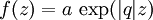$f(z) = a \, \exp(|q| z)$, with some constant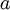$a$. The less trivial condition is the matching between$\psi$ and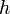$h$: the potential field must correspond to a velocity field that is adjusted to the movement of the surface:$v_z (z=h) =\partial h/\partial t$. This means that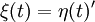$\xi(t)=\eta(t)'$, and that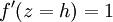$f'(z=h) = 1$, so that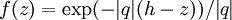$f(z) = \exp( -|q|(h-z))/|q|$. We may now find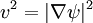$v^2=|\nabla\psi|^2$, which is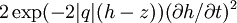$2 \exp( -2 |q|(h-z)) (\partial h/\partial t)^2$. Performing the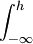$\int_{-\infty}^h$ integration first we are left with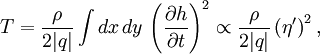$T=\frac{\rho}{2|q|} \int dx\,dy\, \left( \frac{\partial h}{\partial t} \right)^2 \propto \frac{\rho}{2|q|} \left( \eta' \right)^2,$

where we have dropped a factor of$A/4$ in the last step.

The problem is thus specified by just a potential energy involving the square of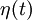$\eta(t)$ and a kinetic energy involving the square of its time derivative: a regular harmonic oscillator. Its equation of motion will be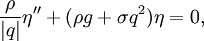$\frac{\rho}{|q|} \eta'' + (\rho g+ \sigma q^2) \eta=0,$

whose oscillatory solution is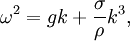$\omega^2=g k+\frac{\sigma}{\rho}k^3,$

the same dispersion as above if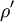$\rho'$ is neglected.